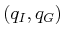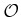#### Notions of completeness

It is useful to define several notions of completeness for sampling-based algorithms. These algorithms have the drawback that they result in weaker guarantees that the problem will be solved. An algorithm is considered complete if for any input it correctly reports whether there is a solution in a finite amount of time. If a solution exists, it must return one in finite time. The combinatorial motion planning methods of Chapter 6 will achieve this. Unfortunately, such completeness is not achieved with sampling-based planning. Instead, weaker notions of completeness are tolerated. The notion of denseness becomes important, which means that the samples come arbitrarily close to any configuration as the number of iterations tends to infinity. A deterministic approach that samples densely will be called resolution complete. This means that if a solution exists, the algorithm will find it in finite time; however, if a solution does not exist, the algorithm may run forever. Many sampling-based approaches are based on random sampling, which is dense with probability one. This leads to algorithms that are probabilistically complete, which means that with enough points, the probability that it finds an existing solution converges to one. The most relevant information, however, is the rate of convergence, which is usually very difficult to establish.

Section 5.1 presents metric and measure space concepts, which are fundamental to nearly all sampling-based planning algorithms. Section 5.2 presents general sampling concepts and quality criteria that are effective for analyzing the performance of sampling-based algorithms. Section 5.3 gives a brief overview of collision detection algorithms, to gain an understanding of the information available to a planning algorithm and the computation price that must be paid to obtain it. Section 5.4 presents a framework that defines algorithms which solve motion planning problems by integrating sampling and discrete planning (i.e., searching) techniques. These approaches can be considered single query in the sense that a single pair,, is given, and the algorithm must search until it finds a solution (or it may report early failure). Section 5.5 focuses on rapidly exploring random trees (RRTs) and rapidly exploring dense trees (RDTs), which are used to develop efficient single-query planning algorithms. Section 5.6 covers multiple-query algorithms, which invest substantial preprocessing effort to build a data structure that is later used to obtain efficient solutions for many initial-goal pairs. In this case, it is assumed that the obstacle regionremains the same for every query.

Steven M LaValle 2020-08-14